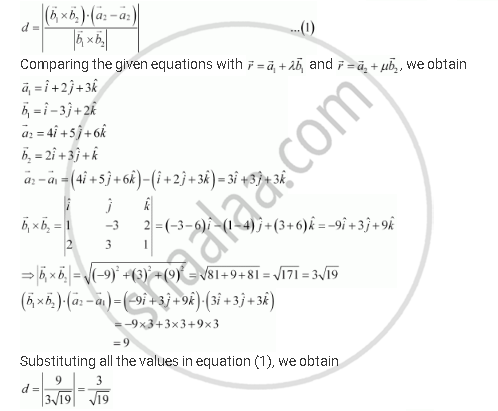Share

# Find the Shortest Distance Between the Lines Whose Vector Equations Are Vecr = (Hati + 2hatj + 3hatk) + Lambda(Hati - 3hatj + 2hatk) and Vecr = 4hati + 5hatj + 6hatk + Mu(2hati + 3hatj + Hatk) - CBSE (Science) Class 12 - Mathematics

ConceptShortest Distance Between Two Lines

#### Question

Find the shortest distance between the lines whose vector equations are vecr = (hati + 2hatj + 3hatk) + lambda(hati - 3hatj + 2hatk) and vecr = 4hati + 5hatj + 6hatk + mu(2hati + 3hatj + hatk)

#### Solution

The given lines are   vecr = (hati + 2hatj + 3hatk) + lambda(hati - 3hatj + 2hatk) and vecr = 4hati + 5hatj + 6hatk + mu(2hati + 3hatj + hatk)

It is known that the shortest distance between the lines, vecr = veca_1 + lambdavecb_1 and ,vecr = veca_2 + muvecb_2 is given by,Therefore, the shortest distance between the two given lines is 3/sqrt19 units

Is there an error in this question or solution?

#### APPEARS IN

NCERT Solution for Mathematics Textbook for Class 12 (2018 to Current)
Chapter 11: Three Dimensional Geometry
Q: 16 | Page no. 478

#### Video TutorialsVIEW ALL 

Solution Find the Shortest Distance Between the Lines Whose Vector Equations Are Vecr = (Hati + 2hatj + 3hatk) + Lambda(Hati - 3hatj + 2hatk) and Vecr = 4hati + 5hatj + 6hatk + Mu(2hati + 3hatj + Hatk) Concept: Shortest Distance Between Two Lines.
S